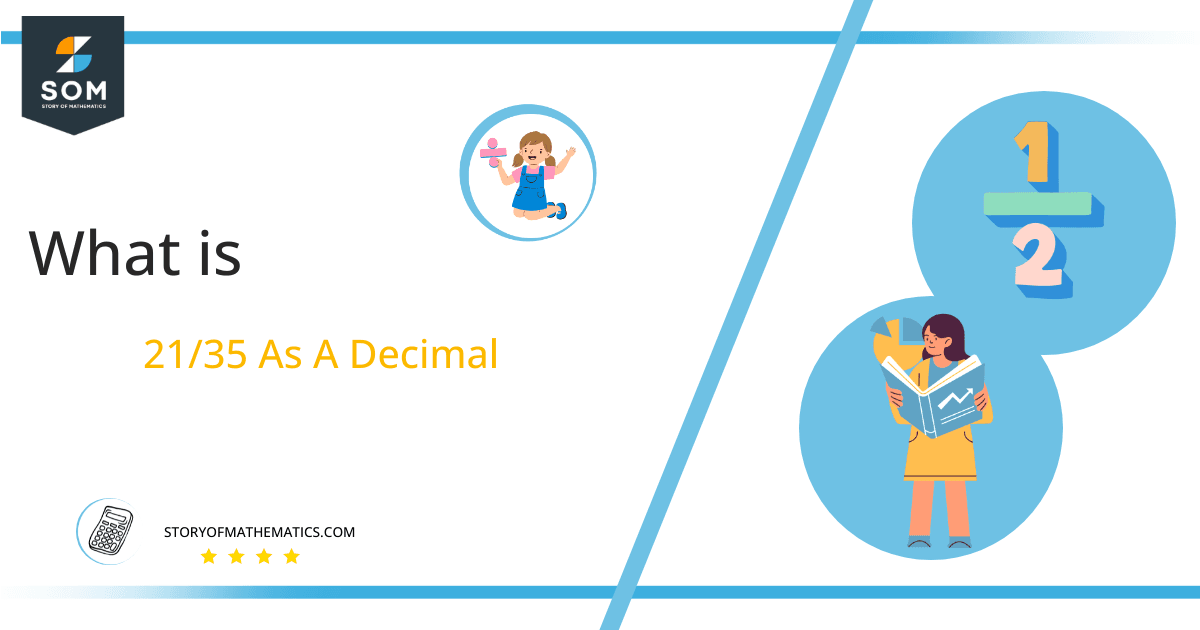# What Is 21/35 as a Decimal + Solution With Free Steps

The fraction 21/35 as a decimal is equal to 0.6.

The fractions can be converted to their decimal forms using the long division method. The decimal form makes the problems easier to solve thus it is required in most of the problems.

Here, we are more interested in the division types that result in a Decimal value, as this can be expressed as a Fraction. We see fractions as a way of showing two numbers having the operation of Division between them that result in a value that lies between two Integers.Now, we introduce the method used to solve said fraction to decimal conversion, called Long Division, which we will discuss in detail moving forward. So, let’s go through the Solution of fraction 21/35.

## Solution

First, we convert the fraction components, i.e., the numerator and the denominator, and transform them into the division constituents, i.e., the Dividend and the Divisor, respectively.

This can be done as follows:

Dividend = 21

Divisor = 35

Now, we introduce the most important quantity in our division process: the Quotient. The value represents the Solution to our division and can be expressed as having the following relationship with the Division constituents:

Quotient = Dividend $\div$ Divisor = 21 $\div$ 35

This is when we go through the Long Division solution to our problem. The solution is given in the figure below.Figure 1

## 21/35 Long Division Method

We start solving a problem using the Long Division Method by first taking apart the division’s components and comparing them. As we have 21 and 35, we can see how 21 is Smaller than 35, and to solve this division, we require that 21 be Bigger than 35.

This is done by multiplying the dividend by 10 and checking whether it is bigger than the divisor or not. If so, we calculate the Multiple of the divisor closest to the dividend and subtract it from the Dividend. This produces the Remainder, which we then use as the dividend later.

Now, we begin solving for our dividend 21, which after getting multiplied by 10 becomes 210.

We take this 210 and divide it by 35; this can be done as follows:

210 $\div$ 35 = 6

Where:

35 x 6 = 210

This will lead to the generation of a Remainder equal to 210 – 210 = 0. As the remainder achieved is equal to zero, therefore there is no need for further long division.

Finally, we have a Quotient generated after combining the one piece of it as 0.6, with a Remainder equal to 0.Images/mathematical drawings are created with GeoGebra.